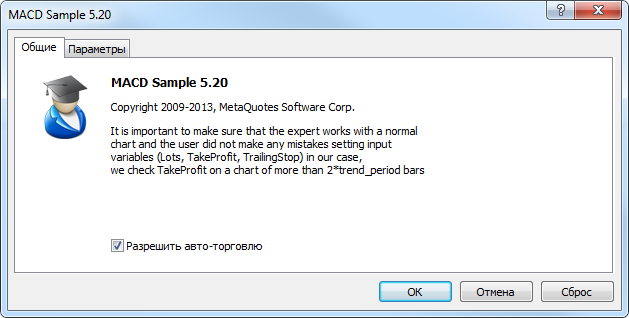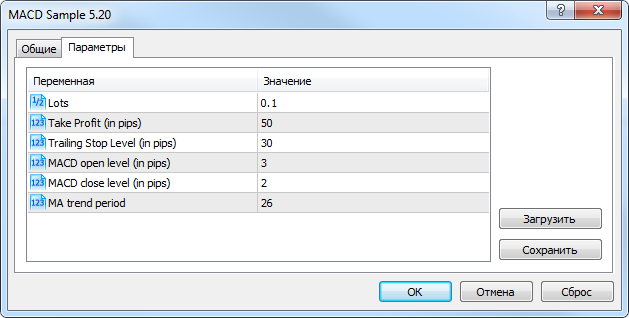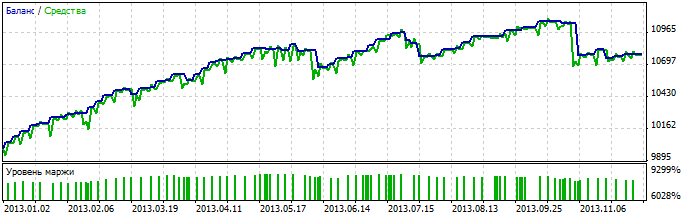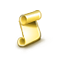Interesting script?
So post a link to it -
let others appraise it
You liked the script? Try it in the MetaTrader 5 terminal# MACD Sample - expert for MetaTrader 5

MetaQuotes Software Corp. |
Views:
39456
Rating:
(51)
Published:
2014.06.02 11:22
Updated:
2016.11.22 07:32

The MACD Sample EA is included in the standard pack of the MetaTrader 5 client terminal and is an example of the EA that trades using the MACD indicator.

The file of the MACD Sample.mq5 expert Advisor is located in terminal_data_folder\MQL5\Experts\Examples\MACD\". This Expert Advisor is an example of object-oriented approach in EA development.

Let's consider the structure of the Expert Advisor and how it works.

1 EA Properties

1.1. EA Properties

```//+------------------------------------------------------------------+
//|                                                  MACD Sample.mq5 |
//|                   Copyright 2009-2013, MetaQuotes Software Corp. |
//|                                              https://www.mql5.com |
//+------------------------------------------------------------------+
#property version     "5.20"
#property description "It is important to make sure that the expert works with a normal"
#property description "chart and the user did not make any mistakes setting input"
#property description "variables (Lots, TakeProfit, TrailingStop) in our case,"
#property description "we check TakeProfit on a chart of more than 2*trend_period bars"```

First 5 rows contain a comment, the following seven lines set the properties of the MQL5-program (copyright, link, version, description) using the preprocessor directives #property.

When you run the Expert Advisor they are displayed in the "Common" tab:Figure 1 Common parameters of the MACD Sample EA

1.2. Include Files

Next, the #include directive tells the compiler to include the files that contain trade classes of the Standard Library.

• SymbolInfo.mqh (CSymbolInfo - a class for working with the properties of a trading instrument);
• PositionInfo.mqh (CPositionInfo - a class for working with open position properties);
• AccountInfo.mqh (CAccountInfo - a class for working with trade account properties).
```//--- include files

Instances of the appropriate classes are then used as member variables of the CExpert class (Section 3).

1.3. Inputs

Then goes the type, name, default values and a comment. Their role is shown in fig. 2.

```//--- Expert Advisor input parameters
input double InpLots          =0.1; // Lots
input int    InpTakeProfit    =50;  // Take Profit (in pips)
input int    InpTrailingStop  =30;  // Trailing Stop Level (in pips)
input int    InpMACDOpenLevel =3;   // MACD open level (in pips)
input int    InpMACDCloseLevel=2;   // MACD close level (in pips)
input int    InpMATrendPeriod =26;  // MA trend period```

Note that the names of the input parameters have the prefix "Inp". Also note that global variables are prefixed with "Ext". Such an approach variable naming simplifies using a lot of different variables.

InpLots - trade volume, InpTakeProfit and InpTrailingStop determine the Take Profit and Trailing Stop levels,

The text in the comment in the input parameter line, along with default values ​, are ​displayed in the "Options" tab instead of the name of the input parameter:Figure 2. Input parameters of the MACD Sample EA

1.4. Global Variables

Then the global variable ExtTimeOut is declared. It will be used for controlling execution time of trade operations.

```int ExtTimeOut=10; // time (in seconds) between trade operations
```

After declaration of the CSampleExpert class, in line 76 another global variable is declared: ExtExpert - CSampleExpert class instance:

```//--- the ExtExpert global variable
CSampleExpert ExtExpert;```

The ExtExpert object (CSampleExpert class sample) contains the basic logic of the trading strategy (Section 3).

2. Event Handling Functions

Event Handling Functions

2.1. The OnInit() initialization function

The OnInit() function is called once during the first start of the Expert Advisor. Usually in the OnInit() event handler the EA is prepared for operation: input parameters are checked, indicators and parameters are initialized, etc. In the case of critical errors, when further work is meaningless, function is exited with a return code INIT_FAILED.

```//+------------------------------------------------------------------+
//| Expert initialization function                                   |
//+------------------------------------------------------------------+
int OnInit(void)
{
//--- initialization and creation of all the required objects
if(!ExtExpert.Init())
return(INIT_FAILED);
//--- successful initialization
return(INIT_SUCCEEDED);
}
```

In this case, the Init() method of the ExtExpert object is called, which returns true or false depending on preparation of all the objects required for operation (see Section 3.4). In case of an error, OnInit() is exited with a return code INIT_FAILED - it is a correct way to complete the EA/indicator operation in the case of an unsuccessful initialization.

2.2. The OnTick() function

The OnTick() function is called each time a new quote is received for the symbol of the chart, on which the EA runs.

```//+------------------------------------------------------------------+
//| Expert new tick handling function                                |
//+------------------------------------------------------------------+
void OnTick(void)
{
static datetime limit_time=0; // stores the last call time + timeout
//--- do not operate if the required time has not elapsed
if(TimeCurrent()>=limit_time)
{
//--- checking data
if(Bars(Symbol(),Period())>2*InpMATrendPeriod)
{
//--- after the call of the Processing() method increase the value of limit_time by ExtTimeOut
if(ExtExpert.Processing())
limit_time=TimeCurrent()+ExtTimeOut;
}
}
}```

In the OnTick() event handler includes a mechanism for periodic calls of the ExtExpert.Processing() method, which is used for market analysis and trading operations when trading conditions are met.

The time interval between the calls is set by the value of the ExtTimeOut input parameter.

2.3. The OnDeInit() deinitialization function

OnDeInit() is called when an EA is removed from the chart. If a program places graphical objects during operation, they can be removed from the chart.

In these example no deinitialization function is used, no actions are performed.

3. The CSampleExpert class

3.1. The CSampleExpert class

```//+------------------------------------------------------------------+
//| The MACD Sample EA class sample                                  |
//+------------------------------------------------------------------+
class CSampleExpert
{
protected:
//--- protected variables - class members are available only inside the class methods
double            m_adjusted_point;             // a multiplier for 3/5-digit quotes
CSymbolInfo       m_symbol;                     // CSymbolInfo class sample
CPositionInfo     m_position;                   // CPositionInfo class sample
CAccountInfo      m_account;                    // CAccountInfo class sample
//--- indicator handles
int               m_handle_macd;                // the MACD indicator handle
int               m_handle_ema;                 // the Moving Average indicator handle
//--- indicator buffers
double            m_buff_MACD_main[];           // the buffer of the main line of the MACD indicator
double            m_buff_MACD_signal[];         // the buffer of the signal line of the MACD indicator
double            m_buff_EMA[];                 // EMA indicator buffers
//--- current indicator values
double            m_macd_current;
double            m_macd_previous;
double            m_signal_current;
double            m_signal_previous;
double            m_ema_current;
double            m_ema_previous;
//--- levels (in standard points)
double            m_macd_open_level;
double            m_macd_close_level;
double            m_traling_stop;
double            m_take_profit;

public:
//--- constructor
CSampleExpert(void);
//--- destructor
~CSampleExpert(void);
//--- public-methods can be called from outside the class
//--- initialization method
bool              Init(void);
//--- deinitialization method
void              Deinit(void);
//--- method of processing
bool              Processing(void);
protected:
//--- protected-methods can be accessed only inside the class methods
bool              InitIndicators(void);
bool              LongClosed(void);
bool              ShortClosed(void);
bool              LongModified(void);
bool              ShortModified(void);
bool              LongOpened(void);
bool              ShortOpened(void);
};```

The EA class contains declaration of variables (class members) and functions (class methods).

For a more convenient work with variables all class member variables contain the prefix "m_" (member), which indicates that a variable is a class member. Before the declaration of a variable or a method, its type is specified (or return value for functions).

Visibility of class member variables and methods is defined using access modifiers. In class CSampleExpert modifiers protected and public are used. All variables and methods defined in the public section, are public and accessible from the outside. The CSampleExpert class has five such methods:

1. CSampleExpert(void) - a constructor  (called automatically when creating a class instance);
2. ~CSampleExpert(void) - a destructor (called automatically when deleting a class instance);
3. bool Init(void) - initialization method, in which all data required for operation are prepared;
4. void Deinit(void) - method of deinitialization;
5. bool Processing(void) - method of processing.

CSampleExpert class member variables declared with the protected access modifier will be available only inside the CSampleExpert class methods (and child classes).

1. double           m_adjusted_point - multiplier variable for a correct operation with 3/5-digit quotes;
3. CSymbolInfo  m_symbol - CSymbolInfo class sample;
4. CPositionInfo  m_position - СPositionInfo class sample;
5. CAccountInfo  m_account - CAccountInfo class sample;
6. int                 m_handle_macd - a variable for storing the value of the MACD indicator handle.
7. int                 m_handle_ema - a variable for storing the value of the EMA indicator handle;
8. double           m_buff_MACD_main[] - a dynamic array of type double, which is used for requesting the values of the main MACD line;
9. double           m_buff_MACD_signal[] - a dynamic array of type double, which is used for requesting the values of the signal MACD line;
10. double           m_buff_EMA[] - a dynamic array of type double, which is used for requesting the values of the EMA indicator;
11. double           m_macd_current - is used for storing the current value of the main MACD line;
12. double           m_macd_previous - is used for storing the previous value of the main MACD line;
13. double           m_signal_current - is used for storing the current value of the signal MACD line;
14. double           m_signal_previous - is used for storing the previous value of the signal MACD line;
15. double           m_ema_current - is used for storing the current value of the EMA indicator;
16. double           m_ema_previous - is used for storing the previous value of the EMA indicator
17. double           m_macd_open_level,
18. double           m_macd_close_level,
19. double           m_traling_stop,
20. double           m_take_profit - are used for storing the values of price levels (set in input parameters) taking into account the m_adjusted_point multiplier.

CSampleExpert class methods declared with the protected access modifier:

1. bool  InitCheckParameters(const int digits_adjust) - checking correctness of input parameters and initialization of EA parameters;
2. bool  InitIndicators(void) - initialization (creation ) of the MACD and Moving Average indicators;
3. bool  LongClosed(void) - returns true (and closes an open long position) if conditions for closing a long position are met;
4. bool  ShortClosed(void) - returns true (and closes an open short position) if conditions for closing a short position are met;
5. bool  LongModified(void) - returns true (and modifies the Stop Loss price) if conditions for changing the Stop Loss level of an open long position are met;
6. bool  ShortModified(void) - returns true (and modifies the Stop Loss price) if conditions for changing the Stop Loss level of an open short position are met;
7. bool  LongOpened(void) - returns true (and opens a long position) if conditions for opening a long position are met;
8. bool  ShortOpened(void) - returns true (and opens a short position) if conditions for opening a short position are met.

3.2. CSampleExpert Class Constructor

```//+------------------------------------------------------------------+
//| CSampleExpert Class Constructor                                  |
//+------------------------------------------------------------------+
m_handle_macd(INVALID_HANDLE),
m_handle_ema(INVALID_HANDLE),
m_macd_current(0),
m_macd_previous(0),
m_signal_current(0),
m_signal_previous(0),
m_ema_current(0),
m_ema_previous(0),
m_macd_open_level(0),
m_macd_close_level(0),
m_traling_stop(0),
m_take_profit(0)
{
ArraySetAsSeries(m_buff_MACD_main,true);
ArraySetAsSeries(m_buff_MACD_signal,true);
ArraySetAsSeries(m_buff_EMA,true);
}```

Class constructor is called automatically when a class sample object is created. When it is called, default values (in brackets) for class member variables are set and timeseries indexing direction is set for m_buff_MACD_main[], m_buff_MACD_signal[], m_buff_EMA[].

3.3. CSampleExpert Class destructor

```//+------------------------------------------------------------------+
//| CSampleExpert class destructor                                   |
//+------------------------------------------------------------------+
CSampleExpert::~CSampleExpert(void)
{
}```

The CSampleExpert class destructor do not contain any code.

3.4. The Init method of the CSampleExpert class

```//+------------------------------------------------------------------+
//| Initialization and verification of input parameters              |
//+------------------------------------------------------------------+
bool CSampleExpert::Init(void)
{
//--- setting common properties
m_symbol.Name(Symbol());              // symbol
//--- taking into account 3/5-digit quotes
if(m_symbol.Digits()==3 || m_symbol.Digits()==5)
//--- setting the values of the levels taking into account the m_adjusted_point modifier
//--- setting the slippage of 3 points
//---
return(false);
if(!InitIndicators())
return(false);
//--- successful completion
return(true);
}```

In the Init() method, class member variables are initialized and the input parameters are verified.

A call of the Name()method for the m_symbol object (CSymbolInfo class instance) sets the name of the symbol, on which the Expert Advisor runs, then method SetExpertMagicNumber() is called; it sets the value of the EA's magic number for the m_trade object (will be used for trade operations). After that, the Digits() method is used for requesting the symbols's number of digits after the decimal point and, if necessary, the values of the levels are corrected.

Next the SetDeviationInPoints() method of the m_trade object is called, in which the value of the allowed slippage in trade operations is set.

3.5. The InitCheckParameters method of the CSampleExpert class

```//+------------------------------------------------------------------+
//| Checking for input parameters                                    |
//+------------------------------------------------------------------+
{
//--- checking correctness of the Take Profit level
{
printf("Take Profit must be greater than %d",m_symbol.StopsLevel());
return(false);
}
//--- checking correctness of the Trailing Stop level
{
printf("Trailing Stop must be greater than %d",m_symbol.StopsLevel());
return(false);
}
//--- checking correctness of the trade volume
if(InpLots<m_symbol.LotsMin() || InpLots>m_symbol.LotsMax())
{
printf("Lots amount must be in the range from %f to %f",m_symbol.LotsMin(),m_symbol.LotsMax());
return(false);
}
if(MathAbs(InpLots/m_symbol.LotsStep()-MathRound(InpLots/m_symbol.LotsStep()))>1.0E-10)
{
printf("Lots amount is not corresponding with lot step %f",m_symbol.LotsStep());
return(false);
}
//--- show warning if Take Profit<=Trailing Stop
if(InpTakeProfit<=InpTrailingStop)
printf("Warning: Trailing Stop must be less than Take Profit");
//--- successful completion
return(true);
}```

The correctness of the EA input parameters is checked in the InitCheckParameters() method. If any of the parameters is invalid, an appropriate message appears, and the function returns false.

3.6. The InitIndicators() method of the CSampleExpert class

```//+------------------------------------------------------------------+
//| Indicators initialization method                                 |
//+------------------------------------------------------------------+
bool CSampleExpert::InitIndicators(void)
{
//--- creating the MACD indicator
if(m_handle_macd==INVALID_HANDLE)
if((m_handle_macd=iMACD(NULL,0,12,26,9,PRICE_CLOSE))==INVALID_HANDLE)
{
printf("Error creating MACD indicator");
return(false);
}
//--- creating the EMA indicator
if(m_handle_ema==INVALID_HANDLE)
if((m_handle_ema=iMA(NULL,0,InpMATrendPeriod,0,MODE_EMA,PRICE_CLOSE))==INVALID_HANDLE)
{
printf("Error creating EMA indicator");
return(false);
}
//--- successful completion
return(true);
}```

In the InitIndicators() method, the correctness of initial values of the m_handle_macd and m_handle_ema variables is checked (they must be equal to INVALID_HANDLE, since they have been initialized in the constructor), and the technical indicators MACD and Moving Average are created (using the iMACD and iMA functions). If successful, the function returns true, and the indicator handles are saved in the m_handle_macd and m_handle_ema class members.

The handles of the created indicators will then be used for checking the amount of calculated data (BarsCalculated) and obtaining the numerical values ​​(CopyBuffer) of the indicators in the Processing() method.

3.7. The LongClosed() method of the CSampleExpert class

```//+------------------------------------------------------------------+
//| Checking conditions for closing a long position                  |
//+------------------------------------------------------------------+
bool CSampleExpert::LongClosed(void)
{
bool res=false;
//--- Should the position be closed?
if(m_macd_current>0)
if(m_macd_current<m_signal_current && m_macd_previous>m_signal_previous)
if(m_macd_current>m_macd_close_level)
{
//--- closing the position
printf("Long position by %s to be closed",Symbol());
else
printf("Error closing position by %s : '%s'",Symbol(),m_trade.ResultComment());
res=true;
}
//--- returning the result
return(res);
}```

The LongClosed() method returns true (and closes the open long position) if conditions for position closing are met:

1. m_macd_current>0 - the current value of the main line of the MACD indicator is positive (MACD histogram is above the zero line);
2. m_macd_current<m_signal_current && m_macd_previous>m_signal_previous - the main line of the MACD indicator has crossed the signal one downwards.
3. m_macd_current>m_macd_close_level - the current value of the main line of the MACD indicator is greater than m_macd_close_level.

3.8. The ShortClosed() method of the CSampleExpert class

```//+------------------------------------------------------------------+
//| Checking conditions for closing a short position                 |
//+------------------------------------------------------------------+
bool CSampleExpert::ShortClosed(void)
{
bool res=false;
//--- Should the position be closed?
if(m_macd_current<0)
if(m_macd_current>m_signal_current && m_macd_previous<m_signal_previous)
if(MathAbs(m_macd_current)>m_macd_close_level)
{
//--- closing the position
printf("Short position by %s to be closed",Symbol());
else
printf("Error closing position by %s : '%s'",Symbol(),m_trade.ResultComment());
res=true;
}
//--- returning the result
return(res);
}```

The ShortClosed() method returns true (and closes an open short position) if positions for closing a short position are met:

1. m_macd_current<0 - the current value of the main line of the MACD indicator is negative (MACD histogram is below the zero line).
2. m_macd_current>m_signal_current && m_macd_previous<m_signal_previous - the main line of the MACD indicator has crossed the signal one upwards.
3. MathAbs(m_macd_current)>m_macd_close_level - the current value of the main line of the MACD indicator is greater than m_macd_close_level.

3.9. The LongModified() method of the CSampleExpert class

```//+------------------------------------------------------------------+
//| Checking conditions for long position modification               |
//+------------------------------------------------------------------+
bool CSampleExpert::LongModified(void)
{
bool res=false;
//--- checking if Trailing Stop is required
if(InpTrailingStop>0)
{
{
double sl=NormalizeDouble(m_symbol.Bid()-m_traling_stop,m_symbol.Digits());
double tp=m_position.TakeProfit();
if(m_position.StopLoss()<sl || m_position.StopLoss()==0.0)
{
//--- modifying Stop Loss and Take Profit of the position
printf("Long position by %s to be modified",Symbol());
else
{
printf("Error modifying position by %s : '%s'",Symbol(),m_trade.ResultComment());
printf("Modify parameters : SL=%f,TP=%f",sl,tp);
}
res=true;
}
}
}
//--- returning the result
return(res);
}```

The LongModified() method returns true (and modifies the Stop Loss value of the position) if conditions for long position modification are met: If the value of the input InpTrailingStop>0, then the fact of price passing InpTrailingStop points from the open price in the position direction is checked. Next, the value of the new Stop Loss level is calculated and the Stop Loss parameter of the open position is modified.

3.10. The ShortModified method of the CSampleExpert class

```//+------------------------------------------------------------------+
//| Checking conditions for long position modification               |
//+------------------------------------------------------------------+
bool CSampleExpert::ShortModified(void)
{
bool   res=false;
//--- checking if Trailing Stop is required
if(InpTrailingStop>0)
{
{
double tp=m_position.TakeProfit();
if(m_position.StopLoss()>sl || m_position.StopLoss()==0.0)
{
//--- modifying Stop Loss and Take Profit of the position
printf("Short position by %s to be modified",Symbol());
else
{
printf("Error modifying position by %s : '%s'",Symbol(),m_trade.ResultComment());
printf("Modify parameters : SL=%f,TP=%f",sl,tp);
}
res=true;
}
}
}
//--- returning the result
return(res);
}```

The ShortModified() method returns true (and modifies the Stop Loss value of the position) if conditions for short position modification are met: If the value of the input InpTrailingStop>0, then the fact of price passing InpTrailingStop points from the open price in the position direction is checked. Next, the value of the new Stop Loss level is calculated and the Stop Loss parameter of the open position is modified.

3.11. The LongOpened() method of the CSampleExpert class

```//+------------------------------------------------------------------+
//| Check for long position opening                                  |
//+------------------------------------------------------------------+
bool CSampleExpert::LongOpened(void)
{
bool res=false;
//--- checking conditions for opening a long position
if(m_macd_current<0)
if(m_macd_current>m_signal_current && m_macd_previous<m_signal_previous)
if(MathAbs(m_macd_current)>(m_macd_open_level) && m_ema_current>m_ema_previous)
{
double tp   =m_symbol.Bid()+m_take_profit;
//--- checking free margin
printf("We have no money. Free Margin = %f",m_account.FreeMargin());
else
{
//--- opening a long position
printf("Position by %s to be opened",Symbol());
else
{
printf("Open parameters : price=%f,TP=%f",price,tp);
}
}
res=true;
}
//--- returning the result
return(res);
}```

The LongOpened() method returns true (and opens a long position) if conditions for opening a buy position are met:

1. m_macd_current<0 - the current value of the main line of the MACD indicator is negative (MACD histogram is below the zero line);
2. m_macd_current>m_signal_current && m_macd_previous<m_signal_previous - the main line of the MACD indicator has crossed the signal one upwards;
3. MathAbs(m_macd_current)>m_macd_open_level - the current value of the main line of the MACD indicator modulo is greater than m_macd_open_level;
4. m_ema_current>m_ema_previous - ema grows.

When all the conditions are met, free margin is checked (the FreeMarginCheck() method of the CAccountInfo Standard library class) and a long position is opened using the PositionOpen() method of the CTrade class.

3.12. The ShortOpened method of the CSampleExpert class

```//+------------------------------------------------------------------+
//| Check for short position opening                                 |
//+------------------------------------------------------------------+
bool CSampleExpert::ShortOpened(void)
{
bool res=false;
//--- checking conditions for opening a short position
if(m_macd_current>0)
if(m_macd_current<m_signal_current && m_macd_previous>m_signal_previous)
if(m_macd_current>(m_macd_open_level) && m_ema_current<m_ema_previous)
{
double price=m_symbol.Bid();
//--- checking free margin
if(m_account.FreeMarginCheck(Symbol(),ORDER_TYPE_SELL,InpLots,price)<0.0)
printf("We have no money. Free Margin = %f",m_account.FreeMargin());
else
{
//--- opening a short position
printf("Position by %s to be opened",Symbol());
else
{
printf("Error opening SELL position by %s : '%s'",Symbol(),m_trade.ResultComment());
printf("Open parameters : price=%f,TP=%f",price,tp);
}
}
res=true;
}
//--- returning the result
return(res);
}```

The ShortOpened() method returns true (and opens a short position) if conditions for opening a sell position are met:

1. m_macd_current>0 - the current value of the main line of the MACD indicator is positive (MACD histogram is above the zero line);
2. m_macd_current<m_signal_current && m_macd_previous>m_signal_previous - the main line of the MACD indicator has crossed the signal one downwards;
3. m_macd_current>m_macd_open_level - the current value of the main MACD line is greater than m_macd_open_level;
4. m_ema_current<m_ema_previous - ema falls.

When all the conditions are met, free margin is checked (the FreeMarginCheck() method of the CAccountInfo Standard library class) and a short position is opened using the PositionOpen() method of the CTrade class.

3.13 The Processing() method of CSampleExpert class

```//+------------------------------------------------------------------+
//| main function returns true if any position processed             |
//+------------------------------------------------------------------+
bool CSampleExpert::Processing(void)
{
//--- updating quotes
if(!m_symbol.RefreshRates())
return(false);
//--- updating indicator values
if(BarsCalculated(m_handle_macd)<2 || BarsCalculated(m_handle_ema)<2)
return(false);
if(CopyBuffer(m_handle_macd,0,0,2,m_buff_MACD_main)  !=2 ||
CopyBuffer(m_handle_macd,1,0,2,m_buff_MACD_signal)!=2 ||
CopyBuffer(m_handle_ema,0,0,2,m_buff_EMA)         !=2)
return(false);
//--- to simplify work with indicators and for faster access
//--- the current values of the indicators are saved in internal variables (class members)
m_macd_current   =m_buff_MACD_main;
m_macd_previous  =m_buff_MACD_main;
m_signal_current =m_buff_MACD_signal;
m_signal_previous=m_buff_MACD_signal;
m_ema_current    =m_buff_EMA;
m_ema_previous   =m_buff_EMA;
//--- correct market entry is important, but a correct exit is even more important
//--- first check if there is an open position
if(m_position.Select(Symbol()))
{
{
//--- if necessary, we try to close or modify a long position
if(LongClosed())
return(true);
if(LongModified())
return(true);
}
else
{
//--- if necessary, we try to close or modify a short position
if(ShortClosed())
return(true);
if(ShortModified())
return(true);
}
}
//--- no open positions
else
{
//--- check conditions and open a long position if necessary
if(LongOpened())
return(true);
//--- check conditions and open a short position if necessary
if(ShortOpened())
return(true);
}
//--- exit without position processing
return(false);
}```

The Processing() method of the CSampleExpert class is the method of the Expert Advisor. The Processing() method is called in the OnTick() event handler, and time interval between successive calls of this method is monitored (no less than ExtTimeOut seconds) (section 2.2).

By calling the RefreshRates() method of the CSymbolInfo class quotes are updated. The BarsCalculated() function is used for requesting the number of bars, for which indicators MACD and Moving Average are calculated (section 3.6.); if the number of bars is less than 2, exit the function and return false.

Next, the CopyBuffer function call requests the last two values ​​of technical indicators (the main and signal MACD lines and Moving Average values ​); and if the amount of data copied is less than two, then exit the function. After that the values of the indicators from arrays m_buff_MACD_main[], m_buff_MACD_signal[] and m_buff_EMA[] are copied to variables m_macd_current, m_macd_previous, m_signal_current, m_signal_previous, m_ema_current and m_ema_previous.

The next step is working with a position carried out by means of class CPositionInfo of the Standard library. If the Select() method call has returned true, this means that currently there is an open position, its type is determined using the PositionType() method. Further work is carried out in accordance with the type of the open position.

4. Backtesting

The best values ​​of the parameters can be found using the Strategy Tester of the MetaTrader 5 terminal.

Figure 3 shows the results of the Expert Advisor testing for 2013 with default settings.Figure 3. Backtesting results of the MACD Sample Expert Advisor

Conclusions

The MACD Sample Expert Advisor, included in the standard delivery pack of the MetaTrader 5 terminal, is an example of object-oriented approach in EA development.

Translated from Russian by MetaQuotes Ltd.
Original code: https://www.mql5.com/ru/code/2154BackgroundCandle_DigitMacd_HTF

The indicator draws candlesticks of a larger timeframe as color filled rectangles in accordance with the colors of the clouds of the DigitMacd indicator.Exp_DigitMacd

The Exp_DigitMacd EA is based on the signals generated by the DigitMacd oscillator.Japanese Candlestick Patterns

Indicator displays different candlestick patterns on chart. It is possible to change colors and disable alerts.Change Chart and Timeframe

Modified scripts to change Charts(next,previous) and Timeframes(higher,lower) of all charts quickly by hotkeys.